Next: Bibliography Up: Dissertation Oliver Triebl Previous: 8. Conclusions and Outlook

# A. Derivation of the Impact-Ionization Integral

In the following the derivation of the impact-ionization integral from Section 5.1.2 will be given. First, the differntial equation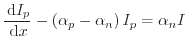(A.1)

has to be solved. Using the simplified notation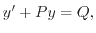(A.2)

with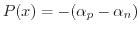andone can derive the homogenous solution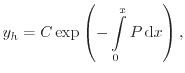(A.3)

where C is the constant of integration. Applying the method of variation of constants, the ansatz of the particular solution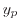is derived from the homogenous solution, using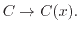This ansatz functioncan be differentiated to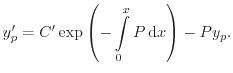(A.4)

Comparison of the coefficients between equations (A.2) and (A.4) gives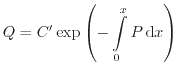(A.5)

and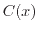evaluates to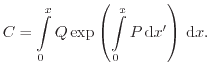(A.6)

This leads to the particular solution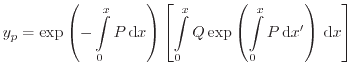(A.7)

and together with the homogenous solution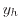to the solution of (A.2)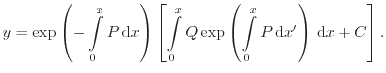(A.8)

Using (A.8) our initial problem (A.1) solves together with the boundary conditions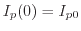and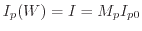to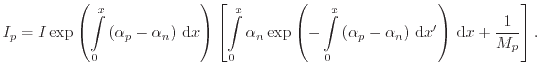(A.9)

To simplify the solution (A.9) the following relationship can be used. Considering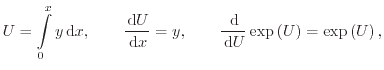(A.10)

the following simplification can be performed: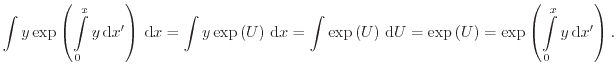(A.11)

Making the relation (A.11) applicable, (A.9) can be rewritten at the position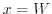as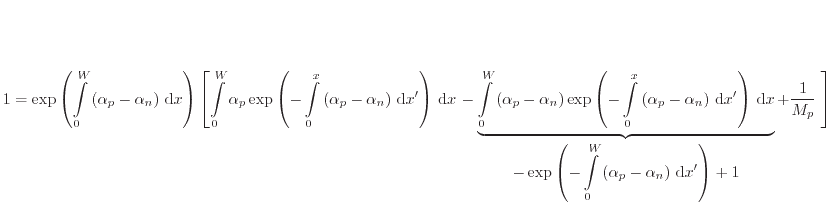(A.12)

and simplified to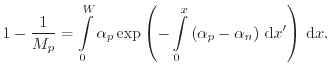(A.13)Next: Bibliography Up: Dissertation Oliver Triebl Previous: 8. Conclusions and Outlook

O. Triebl: Reliability Issues in High-Voltage Semiconductor Devices